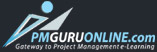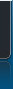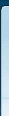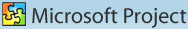Login        |      About MSP     |      Pricing     |     Learning Track     |     Registration     |     FAQ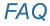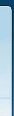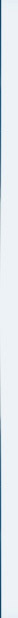Q. 6 What is PERT? Ans. PERT is PROGRAM EVALUATION REVIEW TECHNIQUE. Sometimes in a project one  might be performing the task for the first time. The PERT  model will give an approximate value of duration for a task by considering Optimistic Duration, Expected Duration and Pessimistic Duration for the same task. The duration will be calculated on the basis of weight age factors. The weight factors are as follows: Optimistic Duration is 1, Expected Duration is 4 and Pessimistic Duration is 1. To arrive at the duration of the task the duration mentioned in Optimistic is multiplied with a weight age factor of 1, Expected is multiplied with a weight age factor of 4 and Pessimistic is multiplied with a weight age factor of 1. You will add up the values of Optimistic + Expected + Pessimistic. The total duration is divided by 6. You will arrive with some duration, which is very near to value defined in the Expected Duration.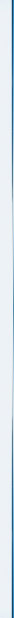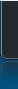Best viewed in 1024x768 resolution in IE 5 and above. | ©2009 pmguruonline.com